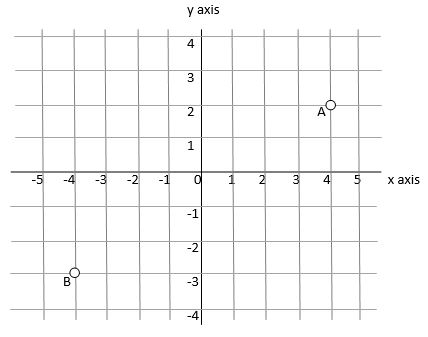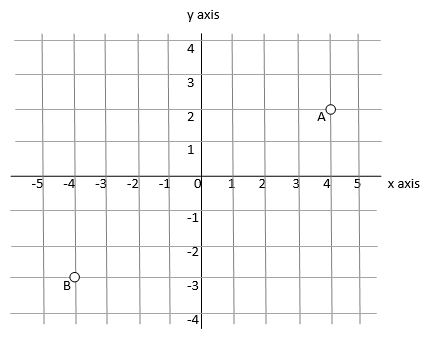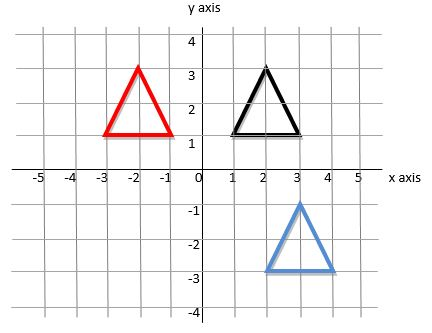# 11-Plus Exam Illustrations - Maths Quiz - Co-ordinates and Translations (Questions)

Co-ordinates

Co-ordinates are used in many applications and in many different ways; for children at 11+ level there is no need to go beyond straightforward 'four quadrant' co-ordinates. In simple terms, this means that they will have to recognise and work with a diagram that looks like this:The idea is that you can locate any point on the diagram using a pair of numbers. The first number of the pair will tell you how far to move along the 'x-axis' (the horizontal line). The second number tells you how far to move along the 'y-axis' (the vertical line). All numbers are in relation to the centre of the diagram, or the 'origin', which is the point marked '0'.

For example, point A in the diagram above is found where the line marking '4' on the x-axis crosses the line marking '2' on the y-axis. That means it is at point (4, 2). Co-ordinates are always put in brackets with a comma in between them.

Point B is to the left and down of the origin, so instead of counting in positive numbers, we have to count in negative numbers. It is crucial that your child understands the idea of negative numbers to handle co-ordinates accurately. Point B is four spaces to the left of the origin, on the line marked '-4'. It is also three spaces down from the origin on the line marked '-3' so the co-ordinates for it are (-4, -3)

A crucial point to note is that the reading MUST work from the horizontal 'x-axis' first. Teachers will refer to 'walking before you fly' or 'going along the corridor then going up the stairs'. This helps children recall that you always look for the horizontal reading before the vertical one. Putting the figures the other way around will give a totally different point.

It is also worth remembering that the co-ordinates may have decimal points in them as well - you can refer to the middle of a square by using, for example, (3.5, 2.5) rather than the point at which the lines cross.

Translations

The mathematical term 'translation' refers to co-ordinate grids and moving shapes around by given measurements. It is a visual-based skill but is also possible to calculate using numbers alone.Let's return to the diagram that we had in the 'co-ordinates' section. The point 'A' is at the co-ordinates (4, 3) but if we want to move it we can translate it. In simple terms, we are going to move it to the left, right, up or down by a given number of squares.

When translating we think of any moves to the right or up as 'positive' and any move to the left or down as being 'negative'. This may be relevant to questions at this stage although most translations will simply be referred to as 'so many places to the left' or something similar.

So, we have point A and want to move it. If we were to move it two places to the left and one place up, it would move to (2, 3). Remember - to read the co-ordinate you look at which numbered lines it sits on, reading the horizontal one first and the vertical one second.

Children are often asked to translate whole shapes.Children may get asked to move the shape themselves or they may be given a second shape and be asked to explain what the translation was that brought it there from the original position.

For example, the black triangle, when moved four places to the left, will end up where the red triangle is. The co-ordinates can be read off from the grid and the move can be done visually but there is a mathematical way to do it too.

The co-ordinates of the black triangle - its three corners - are (1, 1), (3, 1) and (2, 3).

The co-ordinates of a similar triangle moved four places to the left are easily worked out. Four places to the left means the first figure of the co-ordinate, the 'x-axis' value, will be 4 less than it was. The second figure won't change - it's only being moved to the left and not up or down.

The new co-ordinates of the red triangle will be (-3, 1), (-1, 1) and (-2, 3) and these have all been calculated by taking 4 from the first figure in each co-ordinate.

The translation of the black triangle to the blue triangle can be worked out by looking at one point of the black triangle, and counting the squares to the same point on the blue triangle. Count the number of squares in one direction (along) and the number of squares in the other (up or down). The Blue triangle has been made by a translation of one square to the right and four squares down.